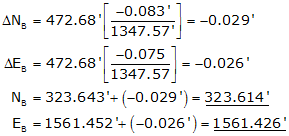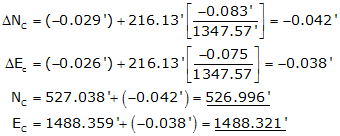## C. Examples

The traverse examples here are the same ones used as previous computation examples so we can check these against earlier results.

### 1. Bearing TraverseFigure I-5 Bearing Traverse Example

Previously computed unadjusted latitudes and departures are:

 Line Bearing Length (ft) Lat (ft) Dep (ft) AB S 68°05'35"W 472.68 -176.357 -438.548 BC N 19°46'00"W 216.13 +203.395 -73.093 CD N 45°55'20"E 276.52 +192.357 +198.651 DA S 54°59'15"E 382.24 -219.312 +313.065 sum: 1347.57 +0.083 +0.075

#### (a) Compute preliminary coordinates from the raw latitudes and departures:#### (b) Determine latitude and departure closure errors:

Lat err = NA' - NA = 500.083' - 500.000' = +0.083'

Dep err = EA' - EA = 2000.075' - 2000.000' = +0.075'

Note that these match the latitude and departure column sums above.

#### (c) Compute and apply corrections to the coordinates using the Compass Rule, Equation (I-3).

Line AB

Because point A is a control point, its coordinates are not modified. The corrections are applied to the line's endpoint, point B.Line BC

Remember to include the corrections for the previous line.Line CDLine DAThe corrected preliminary coordinates match the beginnnig coordinates of point A.

These coordinates also match those computed in the Coordinates section.Figure I-6 Link Traverse Example

Previously computed unadjusted latitudes and departures are:

 Line Direction Length Lat Dep QR S 56°23'38"E 398.75' -220.700' +332.104' RS S 75°17'42"W 422.89' -107.347' -409.038 ST N 43°05'47"E 604.49' +441.402' +413.004' sums: 1426.13' +113.355' +336.070

#### (a) Compute preliminary coordinates:

 Point North (ft) East (ft) Q 2600.480 1391.670 LatQR -220.700 DepQR +332.104 R 2379.780 1723.774 LatRS -107.347 DepRS -409.038 S 2272.433 1314.736 LatST +441.402 DepST +413.004 T 2713.835 1727.740

Lat err = 2713.835' - 2713.780' = +0.555'

Dep err = 1727.740'-1727.810' = -0.070'

#### (c) Compute and apply corrections to the coordinates using the Compass Rule, Equation I-3.Computations for each line are not shown, but their results are tabulated below.

 Point N' (ft) ΔN (ft) N (ft) E' (ft) ΔE (ft) E (ft) S 2379.780 -0.015 2379.765 1723.774 +0.020 1723.794 T 2272.433 -0.031 2272.402 1314.736 +0.041 1314.777 Q 2713.835 -0.054 2713.781 1727.740 +0.071 1727.811 check check